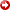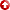[First Hit]Model of Near-Surface Velocity, Southern San Joaquin Valley, California

James G. Ferry

A model was developed that accounts for temporal and spatial variations in the near-surface velocity of the southern San Joaquin Valley. Significant temporal variations intraveltime-vs.-depth measurements have been caused by changing water-table levels over the last 50 years. Spatial velocity variations, at any particular point in time, are due to spatial variations in geology and water-table depth. A theoreticaltraveltimeequation was fit to measurements oftraveltime-vs.-depth and water-table depth. Thistraveltimeequation was derived from the velocity model where z is the depth below the surface, Dw is the depth of the water table, V is the velocity at one unit depth, Vw is the velocity below the water table, and B is the inverse-power coefficient. Fitting all of the data from the area, an inverse model with parameters V = 268 m/sec, B = 2.60, and Vw = 2,170 m/sec was determined with an RMStraveltimeerror of 17 m/sec. Inverse models were determined with RMStraveltimeerrors of 10 m/sec or less when the theoretical model was fit to subsets of data from areas of similar geologic conditions. The sea level datum correctional traveltimes calculated using the inverse models were found to correlate with actualtraveltimemeasurements much better than static corrections used in the past. The inverse model acco nts for false time-structure as great as 180 m/sec in seismic-reflection data that has been static-corrected using a sea level datum correctional velocity of 1,830 m/sec.

AAPG Search and Discovery Article #91024©1989 AAPG Pacific Section, May 10-12, 1989, Palm Springs, California.